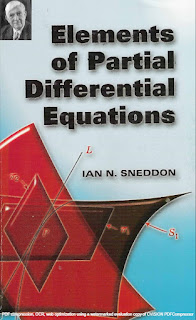DISCRETE MATHEMATICS

# Elements of Partial Differential Equations by Ian N. Sneddon PDF Free DownloadGeared toward students of applied rather than pure mathematics, this volume introduces elements of partial differential equations. Its focus is primarily upon finding solutions to particular equations rather than general theory. Topics include ordinary differential equations in more than two variables, partial differential equations of the first and second orders, Laplace’s equation, the wave equation, and the diffusion equation. A helpful Appendix offers information on systems of surfaces, and solutions to the odd-numbered problems appear at the end of the book. Readers pursuing independent study will particularly appreciate the worked examples that appear throughout the text.